Definitions of Square Dance Calls and Concepts

Index -->  Plus  |  A1  |  A2  |  C1  |  C2  |  C3A  |  C3B  |  C4  |  NOL  |
Definitions (Text Only) -->  Plus  |  A1  |  A2  |  C1  |  C2  |  C3A  |  C3B  |  C4  |  NOL  |
 Find call:

 \$B8@8l(B

From a 2 x 4. EN: 10
2 x 4 \$B\$+\$i!%(B JP: 10

Do a total of four Split Circulates, except that when you become an In-facing Center do your next Circulate to your diagonal opposite position (crossing over into the other Box). Exchange The Box can be fractionalized into fourths. EN: 20
Split Circulate \$B\$r9g7W(B 4 \$B2s9T\$\$\$^\$9\$,(B, \$BCf\$r8~\$\$\$?(B Center \$B\$H\$J\$C\$?\$H\$-\$O\$N(B box \$B\$X(B cross over \$B\$7\$^\$9(B)\$B!%(BExchange The Box \$B\$O(B 4 \$B\$D\$N%Q!<%H\$KJ,\$1\$i\$l\$^\$9!%(B JP: 20

Traffic pattern: when crossing to the other Box, stay outside of those who are still in their original Box. The In-facing Centers lead the Exchange and, from a normal setup, everyone else in their Box can follow them. EN: 30
\$BB>\$N(B box \$B\$X(B cross \$B\$9\$k\$H\$-\$NDL\$jJ}\$G\$9\$,(B, \$B\$^\$@<+J,\$N%*%j%8%J%k\$N(B box \$B\$K\$\$\$k?M\$N30B&\$rDL\$j\$^\$9!%Cf\$r8~\$\$\$?(B Centers \$B\$,(B Exchange \$B\$N%j!<%@!<\$H\$J\$j(B, \$BIaDL\$N>l9g(B box \$B\$NB>\$N?M\$O\$=\$N?M\$K\$D\$\$\$F9T\$/\$3\$H\$,\$G\$-\$^\$9!%(B JP: 30

From Parallel Waves (In-Facing Centers lead): EN: 40
\$BJ?9T\$J(B Wave \$B\$+\$i(B (\$BCf\$r8~\$\$\$?(B Centers \$B\$,%j!<%I(B)JP: 40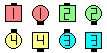Exchange The Box\$B\$NA0(B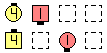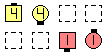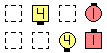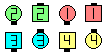the left Boxafter 1/4 EN: 50 \$B:8\$N(B Box1/4 \$B\$N8e(B JP: 50 the left Boxafter 1/2 EN: 60 \$B:8\$N(B Box1/2 \$B\$N8e(B JP: 60 the left Boxafter 3/4 EN: 70 \$B:8\$N(B Box3/4 \$B\$N8e(B JP: 70 \$B8e(B
From Columns (In-Facing Centers lead): EN: 80
Column \$B\$+\$i(B (\$BCf\$r8~\$\$\$?(B Centers \$B\$,%j!<%I(B)JP: 80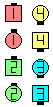Exchange The Box\$B\$NA0(B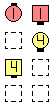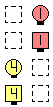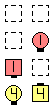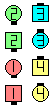the upper Boxafter 1/4 EN: 90 \$B>e\$N(B Box1/4 \$B\$N8e(B JP: 90 the upper Boxafter 1/2 EN: 100 \$B>e\$N(B Box1/2 \$B\$N8e(B JP: 100 the upper Boxafter 3/4 EN: 110 \$B>e\$N(B Box3/4 \$B\$N8e(B JP: 110 \$B8e(B

Dancing hint: Those leading the exchange should raise a hand to identify themselves so that the other dancers in their Boxes can follow them. EN: 120
\$B%@%s%9\$N%R%s%H(B: Exchange \$B\$N%j!<%@!<\$Oe\$2\$F(B, \$BB>\$N?M\$,\$D\$\$\$F9T\$1\$k\$h\$&\$K\$7\$^\$9!%(B JP: 120

\$BExchange The Triangle [C3A].CALLERLAB definition for Exchange The BoxChoreography for Exchange The BoxComments? Questions? Suggestions?

https://www.ceder.net/def/exchangethebox.php?level=C3A&language=japan&action=edit
28-June-2022 10:49:20